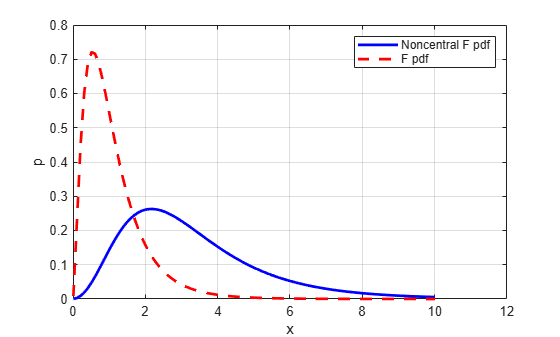Main Content

## Noncentral F Distribution

### Definition

Similar to the noncentral χ2 distribution, the toolbox calculates noncentral F distribution probabilities as a weighted sum of incomplete beta functions using Poisson probabilities as the weights.

`$F\left(x|{\nu }_{1},{\nu }_{2},\delta \right)=\sum _{j=0}^{\infty }\left(\frac{{\left(\frac{1}{2}\delta \right)}^{j}}{j!}{e}^{\frac{-\delta }{2}}\right)I\left(\frac{{\nu }_{1}\cdot x}{{\nu }_{2}+{\nu }_{1}\cdot x}|\frac{{\nu }_{1}}{2}+j,\frac{{\nu }_{2}}{2}\right)$`

I(x|a,b) is the incomplete beta function with parameters a and b, and δ is the noncentrality parameter.

### Background

As with the χ2 distribution, the F distribution is a special case of the noncentral F distribution. The F distribution is the result of taking the ratio of χ2 random variables each divided by its degrees of freedom.

If the numerator of the ratio is a noncentral chi-square random variable divided by its degrees of freedom, the resulting distribution is the noncentral F distribution.

The main application of the noncentral F distribution is to calculate the power of a hypothesis test relative to a particular alternative.

### Examples

#### Compute Noncentral F Distribution pdf

Compute the pdf of a noncentral F distribution with degrees of freedom `NU1 = 5` and `NU2 = 20`, and noncentrality parameter `DELTA = 10`. For comparison, also compute the pdf of an F distribution with the same degrees of freedom.

```x = (0.01:0.1:10.01)'; p1 = ncfpdf(x,5,20,10); p = fpdf(x,5,20);```

Plot the pdf of the noncentral F distribution and the pdf of the F distribution on the same figure.

```figure; plot(x,p1,'b-','LineWidth',2) hold on plot(x,p,'g--','LineWidth',2) legend('Noncentral F','F distribution')```Download ebook